Function Repository Resource:

# FromNumberExpansion

Rational approximation of a real number using different kinds of commonly known series expansions

Contributed by: Swastik Banerjee
 ResourceFunction["FromNumberExpansion"][list,"t"] reconstructs a number from the list of its series expansion terms for the chosen expansion type t.

## Details and Options

"Engel", "Pierce", "Sylvester", "Cantor", "CantorProduct", "Lüroth" and "Oppenheim" are supported as the last argument to specify the type of expansion.
"Lüroth" can also be written as "Lueroth" when passed as an argument.
ResourceFunction["FromNumberExpansion"][{a1,a2,a3,},"Engel"] returns 1/a1+1/a1a2+1/a1a2a3+.
ResourceFunction["FromNumberExpansion"][{a1,a2,a3,},"Pierce"] returns 1/a1-1/a1a2+1/a1a2a3-.
ResourceFunction["FromNumberExpansion"][{a1,a2,a3,},"Sylvester"] returns 1/a1+1/a2+1/a3+.
ResourceFunction["FromNumberExpansion"][{a1,a2,a3,},"Cantor"] returns a1*1!+a2*2!+a3*3!+.
ResourceFunction["FromNumberExpansion"][{a1,a2,a3,},"CantorProduct"] returns (1+1/a1)(1+1/a2)(1+1/a3).
ResourceFunction["FromNumberExpansion"][{a1,a2,a3,},"Lüroth"] returns 1/a1 + 1/a1(a1-1)a2 + 1/a1(a1-1)a2(a2-1)a3+.
ResourceFunction["FromNumberExpansion"][{d1,d2,d3,},r,s,p,q,"Oppenheim"] returns 1/d1+(a1/b1)(1/d2)+(a1a2/b1b2)(1/d2)+, where ai=r+s*di and bi=p+q*di for i=1,2,.,n.
ResourceFunction["FromNumberExpansion"][{p,{a1,a2,a3,,ap}},"Lüroth"] returns an approximation of the rational number whose Lüroth expansion is an infinite periodic series with periodicity p.
ResourceFunction["FromNumberExpansion"] acts as the inverse of the resource function NumberExpansion.

## Examples

### Basic Examples (1)

Rational approximation of the number π using FromNumberExpansion when the series expansion of the number is known:

 In:=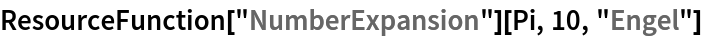Out=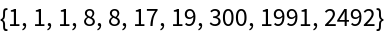In:=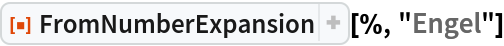Out=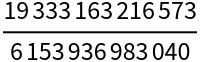In:=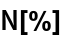Out=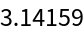### Scope (2)

Approximation of a Real number whose Lüroth series has infinite periodic expansion:

 In:=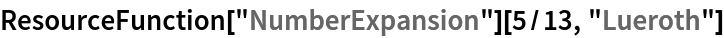Out=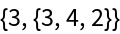In:=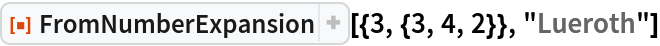Out=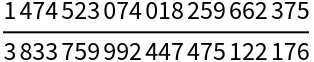In:=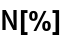Out=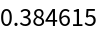In:=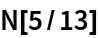Out=Symbolic form:

 In:=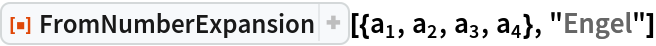Out=### Applications (2)

Rational approximation of an irrational number through different kinds of series expansion representations:

 In:=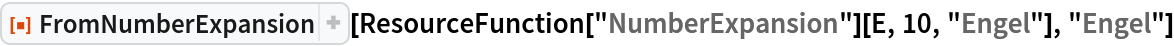Out=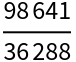In:=Out=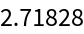Rational approximation of an infinite series:

 In:=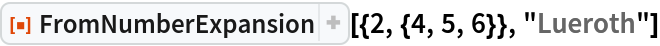Out=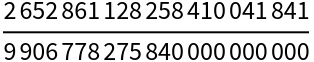## Publisher

Wolfram Summer School

## Version History

• 1.0.0 – 14 September 2020